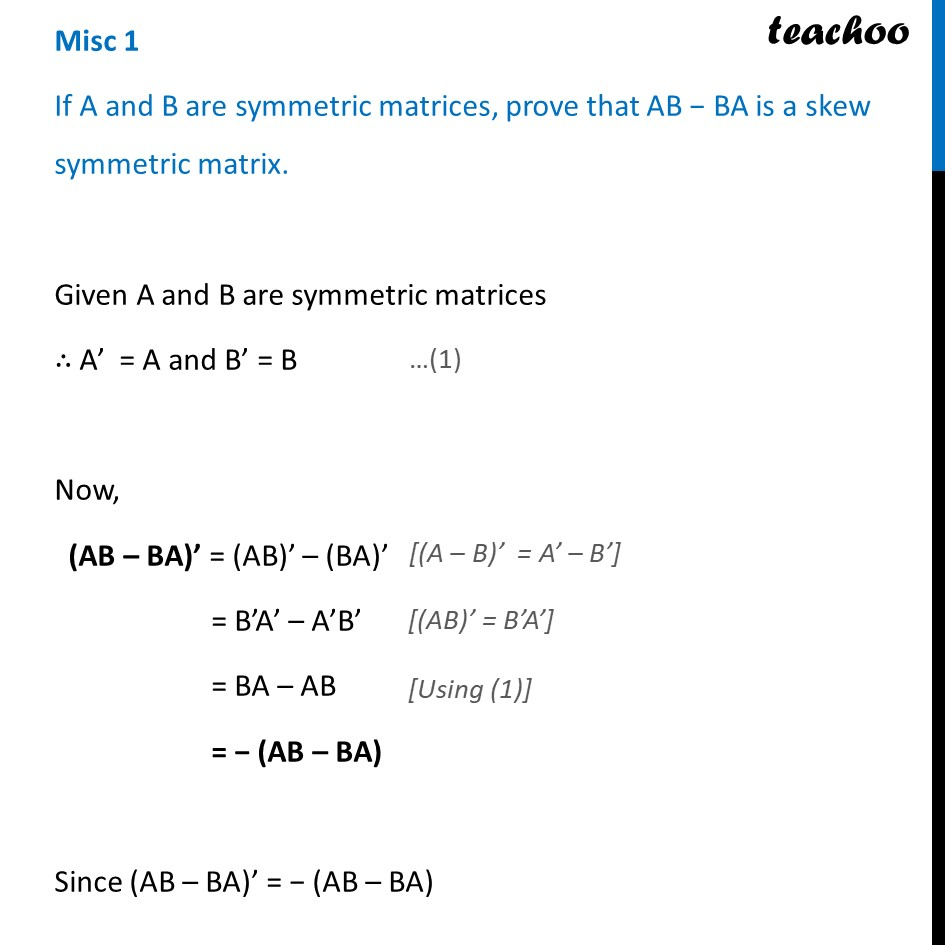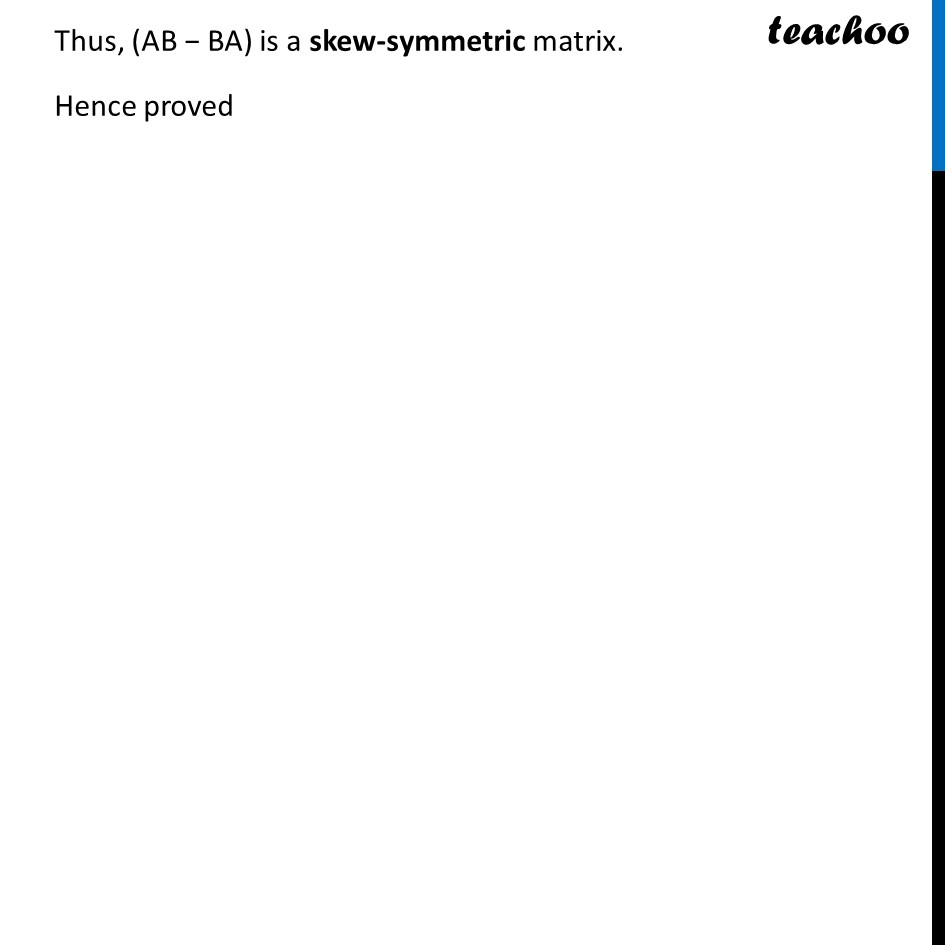Miscellaneous

Chapter 3 Class 12 Matrices
Serial order wiseLearn in your speed, with individual attention - Teachoo Maths 1-on-1 Class

### Transcript

Misc 1 If A and B are symmetric matrices, prove that AB − BA is a skew symmetric matrix. Given A and B are symmetric matrices ∴ A’ = A and B’ = B Now, (AB – BA)’ = (AB)’ – (BA)’ = B’A’ – A’B’ = BA – AB = − (AB – BA) Since (AB – BA)’ = − (AB – BA) Thus, (AB − BA) is a skew-symmetric matrix. Hence proved